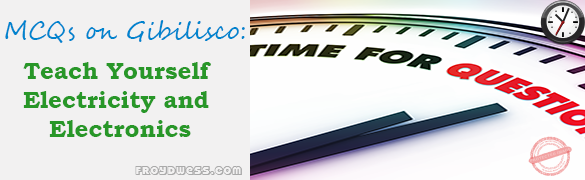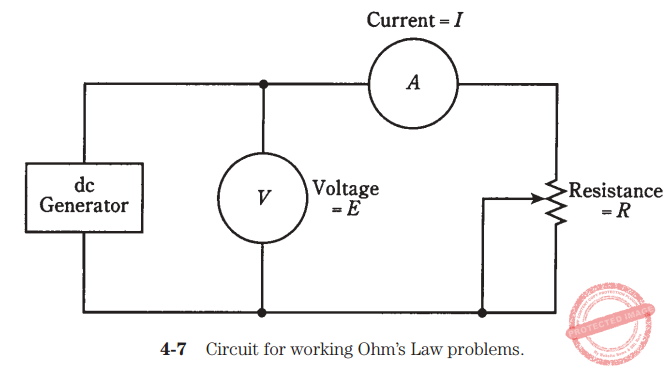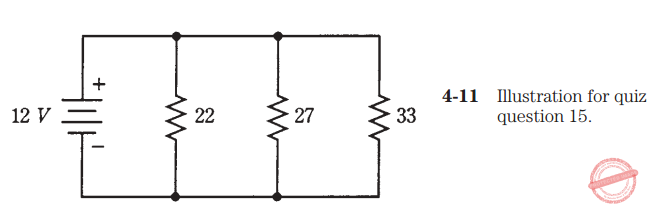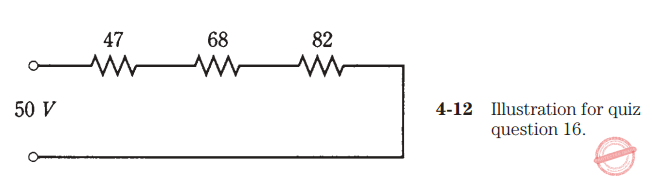# Basic DC Circuits – Gibilisco MCQs in Electronics

(Last Updated On: December 8, 2017)This is the Multiple Choice Questions (MCQs) in Chapter 4: Basic DC Circuits from the book Teach Yourself Electricity and Electronics, 5th edition by Stan Gibilisco. If you are looking for a reviewer in Electronics Engineering this will definitely help you before taking the Board Exam.

### Start Practice Exam Test questions

1. Suppose you double the voltage in a simple dc circuit, and cut the resistance in half. The current will become:

• A. Four times as great.
• B. Twice as great.
• C. The same as it was before.
• D. Half as great.

2. A wiring diagram would most likely be found in:

• A. An engineer’s general circuit idea notebook.
• B. An advertisement for an electrical device.
• C. The service/repair manual for a radio receiver.
• D. A procedural flowchart.

NOTE: For questions 3 through 11, see Fig. 4-7.3. Given a dc voltage source delivering 24 V and a circuit resistance of 3.3 KΩ, what is the current?

• A. 0.73 A.
• B. 138 A.
• C. 138 mA.
• D. 7.3 mA.

4. Suppose that a circuit has 472 Ω of resistance and the current is 875 mA. Then the source voltage is:

• A. 413 V.
• B. 0.539 V.
• C. 1.85 V.
• D. None of the above.

5. The dc voltage in a circuit is 550 mV and the current is 7.2 mA. Then the resistance is:

• A. 0.76 Ω.
• B. 76 Ω.
• C. 0.0040 Ω.
• D. None of the above.

6. Given a dc voltage source of 3.5 kV and a circuit resistance of 220 Ω, what is the current?

• A. 16 mA.
• B. 6.3 mA.
• C. 6.3 A.
• D. None of the above.

7. A circuit has a total resistance of 473,332 Ω and draws 4.4 mA. The best expression for the voltage of the source is:

• A. 2082 V.
• B. 110 kV.
• C. 2.1 kV.
• D. 2.08266 kV.

8. A source delivers 12 V and the current is 777 mA. Then the best expression for the resistance is:

• A. 15 Ω.
• B. 15.4 Ω.
• C. 9.3 Ω.
• D. 9.32 Ω.

9. The voltage is 250 V and the current is 8.0 mA. The power dissipated by the potentiometer is:

• A. 31 mW.
• B. 31 W.
• C. 2.0 W.
• D. 2.0 mW.

10. The voltage from the source is 12 V and the potentiometer is set for 470 Ω. The power is about:

• A. 310 mW.
• B. 25.5 mW.
• C. 39.2 W.
• D. 3.26 W.

11. The current through the potentiometer is 17 mA and its value is 1.22KΩ. The power is:

• A. 0.24 μW.
• B. 20.7 W.
• C. 20.7 mW.
• D. 350 mW.

12. Suppose six resistors are hooked up in series, and each of them has a value of 540 Ω. Then the total resistance is:

• A. 90 Ω.
• B. 3.24 KΩ.
• C. 540 Ω.
• D. None of the above.

13. Four resistors are connected in series, each with a value of 4.0 KΩ. The total resistance is:

• A. 1 KΩ.
• B. 4 KΩ.
• C. 8 KΩ.
• D. 16 KΩ.

14. Suppose you have three resistors in parallel, each with a value of 68,000 Ω. Then the total resistance is:

• A. 23 Ω.
• B. 23 KΩ.
• C. 204 Ω.
• D. 0.2 MΩ.

15. There are three resistors in parallel, with values of 22 Ω, 27Ω, and 33 Ω. A 12-V battery is connected across this combination, as shown in Fig. 4-11. What is the current drawn from the battery by this resistance combination?• A. 1.3 A.
• B. 15 mA.
• C. 150 mA.
• D. 1.5 A.

16. Three resistors, with values of 47 Ω, 68 Ω, and 82 Ω, are connected in series with a 50-V dc generator, as shown in Fig. 4-12. The total power consumed by this network of resistors is:• A. 250 mW.
• B. 13 mW.
• C. 13 W.
• D. Not determinable from the data given.

17. You have an unlimited supply of 1-W, 100-Ω resistors. You need to get a 100- Ω, 10-W resistor. This can be done most cheaply by means of a series-parallel matrix of

• A. 3 X 3 resistors.
• B. 4 X 3 resistors.
• C. 4 X 4 resistors.
• D. 2 X 5 resistors.

18. You have an unlimited supply of 1-W, 1000-Ω resistors, and you need a 500-Ω resistance rated at 7 W or more. This can be done by assembling:

• A. Four sets of two 1000-Ω resistors in series, and connecting these four sets in parallel.
• B. Four sets of two 1000-Ω resistors in parallel, and connecting these four sets in series.
• C. A 3 X 3 series-parallel matrix of 1000-Ω resistors.
• D. Something other than any of the above.

19. You have an unlimited supply of 1-W, 1000-Ω resistors, and you need to get a 3000-Ω, 5-W resistance. The best way is to:

• A. Make a 2 X 2 series-parallel matrix.
• B. Connect three of the resistors in parallel.
• C. Make a 3 X 3 series-parallel matrix.
• D. Do something other than any of the above.

20. Good engineering practice usually requires that a series-parallel resistive network be made:

• A. From resistors that are all very rugged.
• B. From resistors that are all the same.
• C. From a series combination of resistors in parallel.
• D. From a parallel combination of resistors in series.

Rate this: